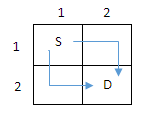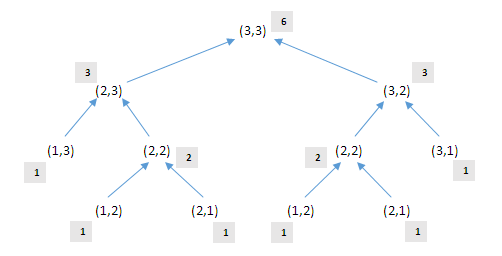# How to traverse a grid or matrix using recursion

 Category: Data Structure And Algorithms Tags:Traversing a Grid Code Files

## Introduction

Suppose a given grid M×N where M are rows and N are columns then we have to find out how many ways are there to move from index (1,1) to (M,N) if we only can move right and down.

## Implementation

Suppose there is grid of 2×2 as shown below then only 2 ways can be there to reach from (1,1) to (M,N) we can not move diagonally only can move right and down.In above picture we have S means source and D means destination and we cannot move diagonally, only we can move right and down so there will be only 2 ways in 2×2 grid.
So same we can achieve if we move from (2,2) to (1,1) in above picture if we move only left and up. If we start from (2,2) so only 2 moves will be there (1,2) and (2,1) and once any one of the coordinate(row and column) reaches to one it has to go only left or right, there will be no any other moves. Now suppose we try to solve with recursion and having grid of 3×3, so:Above picture you can see we are splitting M×N in manner of (M-1, N) and (M, N-1) and wherever any coordinate is 1 we say it is left with only one way (in grey background). When we backtrack the recursion tree and go up we sum the children values and lead to 1+1=2, 2+1=3, 3+3=6. So there will be 6 ways to reach from (1,1) to (3,3) or vice versa if we follow direction rules.
Now lets see it with code:

```static int GetWays(int x, int y)
{
if (x == 1 || y == 1)
return 1;
else
return GetWays(x - 1, y) + GetWays(x, y - 1);
}
```

Above we can see we are returning 1 if any of the coordinate equals to one until that we break it in (M-1, N) and (M, N-1). And we will execute this code from Main method:

```int M = 3;
int N = 3;
Console.WriteLine("Ways of reaching from (1,1) to ({0},{1}) are: {2}", M, N, GetWays(3, 3));
```

And output will be:
Ways of reaching from (1,1) to (3,3) are: 6

 Like1 PersonNikhil Joshi Ceo & Founder at Dotnetlovers Atricles: 146 Questions: 16 Given Best Solutions: 16 *Nice article
Like0 People on 27 August 2017

Login via:x